## Building an alternating current sine wave in Python¶

### Experimenting with wave properties amplitude, wavelength, and frequency¶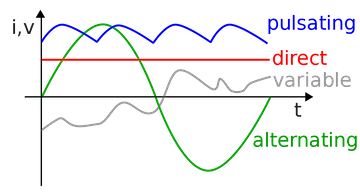Figure 1) In this lesson, we'll be seeing how sine waves represent periodic oscillations, like the green alternating current above. Today's example represents AC current, so the X coordinate is time (t), and the y coordinate is current (i) or voltage (v).

The sine function is a trigonometric function of an angle. Let a line through the origin intersect the unit circle, making an angle of θ with the positive half of the x-axis. The x- and y-coordinates of this point of intersection are equal to cos(θ) and sin(θ), respectively. Remember the unit circle is a circle with a radius of one, and sine and cosine are defined in terms of periodic movement around the circle.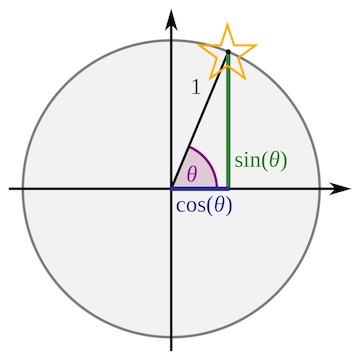Look what happens if we animate our sine function at each point in the circle below. We have a sine wave!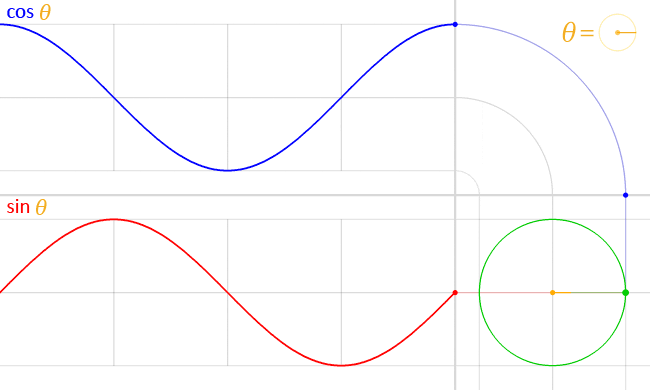## Given what you know about the sine function, what do you think the AMPLITUDE of the wave generated by the sine function is??¶

As you can see, both cosine and sine waves are periodic, which means that after a certain time, the period, they look the same again. Also, both waves look alike, but the cosine wave appears to start at its maximum, while the sine wave starts at zero. Now in practice, how can we tell whether a wave we observe at a given time started out at its maximum, or at zero? Good question: we can’t. There’s no way to discern a sine wave and a cosine wave in practice, thus we call any wave that looks like a sine or cosine wave a “sinusoid”, which is Greek and translates to “sinus-like”. An important property of sinusoids is “frequency”, which tells us how many peaks and valleys we can count in a given period of time. High frequency means many peaks and valleys, low frequency means few peaks and valleys

Now that you know what a sine function is, and how a sine wave represents, let's code one out in Python. Use the np.arange function to choose your sample size (from 0 to 10) and 0.1 your frequency. You can play around with these numbers in the binder too. Amplitude of the sine wave is sine of a variable. We'll use time, which you'll notice we just used in the [In] above. If we were making a sine wave to represent an image instead of alternating current, the x axis variable might be "space" for both the line above and this one. Note: Since the sine function varies from +1 to -1, the amplitude is one.

To get started, we'll use an import statement to call two modules, numpy and matplotlib, that will let us build the sine wave.

In :
import numpy as np
import matplotlib.pyplot as plt
time        = np.arange(0, 10, 0.1);
amplitude = np.sin(time)
plt.plot(time, amplitude)

Out:
[<matplotlib.lines.Line2D at 0x1183359d0>]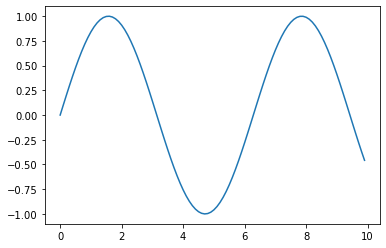An important property of sinusoids is “frequency”, which tells us how many peaks and valleys we can count in a given period of time. High frequency means many peaks and valleys, low frequency means few peaks and valleys. In the code below f is frequency:

In :
Fs = 8000
f = 5
sample = 8000
x = np.arange(sample)
y = np.sin(2 * np.pi * f * x / Fs)
plt.plot(x, y)
plt.xlabel('time(ms)')
plt.ylabel('voltage(V)')
plt.show()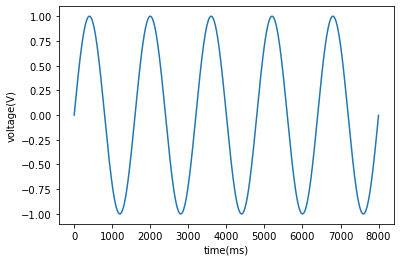In :
fs = 100 # sample rate
f = 6 # the frequency of the signal

x = np.arange(fs) # the points on the x axis for plotting
# compute the value (amplitude) of the sin wave at the for each sample
y = np.sin(2*np.pi*f * (x/fs))

In :
plt.plot(x, y)
plt.xlabel('time(ms)')
plt.ylabel('amplitude is current or voltage for AC')
# showing the exact location of the samples
plt.stem(x,y, 'r', )

Out:
<StemContainer object of 3 artists>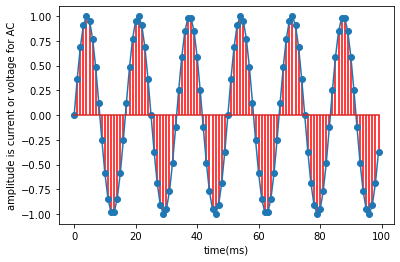In [ ]: